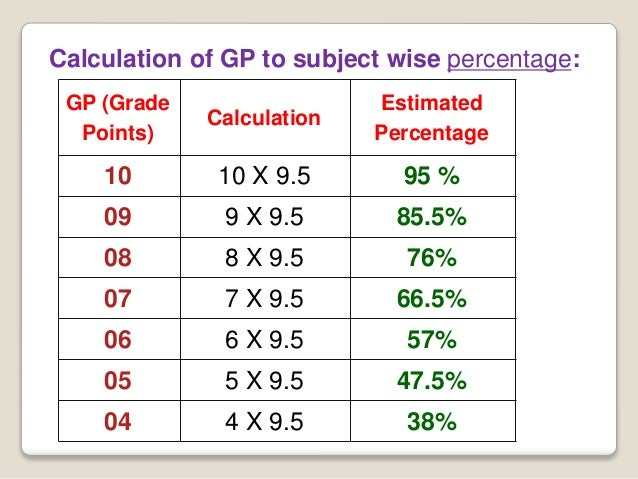# CBSE Class 10 Results 2017: How to calculate CGPA ?

## CBSE Class 10 Results 2017: How to calculate CGPA Cumulative Grade Points Average ?

CBSE Class 10 Results 2017: How to calculate CGPA ? :The results of CBSE Class 10 board exam 2017 will be announced soon at cbse.nic.in. (CBSE board class X results expected to be released at any time from now onwards.CBSE Class 10 Results 2017: How to calculate CGPA
The Cumulative Grade Points Average (CGPA) is the average of grade points achieved in all the subjects (excluding additional 6th subject) as per the scheme of studies.

### What is CGPA ?

CGPA stands for Cumulative Grade Points Average. It is the average of grades in best 5 subjects. CBSE no longer ranks class 10 students on the basis of marks but on the basis of CGPA. It is calculated in a scale of 10, where highest is 10 and lowest is 4.
CBSE swards the students grade points on the basis of marks they obtain in a subject. Here’s the grade chart of CBSE:
91-100 A1 10
81-90 A2 9
71-80 B1 8
61-70 B2 7
51-60 C1 6
41-50 C2 5
33-40 D 4
21-32 E1
00-20 E2
In order to calculate the scores, the candidates can check the example shown below:
Grade Points for 5 subjects are:
Subject 1: 9
Subject 2: 8
subject 3: 7
Subject 4: 10
Subject 5: 9
Step 1: Add the grade points of all the five subjects: 9+8+7+10+9= 43
Step 2: Divide the total by 5: 43/5= 8.6
Overall CGPA=8.6
Step 3: Multiply by 9.5 i.e. 9.5*8.6= 81.7 per cent (overall percentage)
For subject-wise percentage, multiply 9.5 with the Grade Points of that particular subject.

In order to convert your overall CGPA into percentage, multiply it by 9.5.
For example,
In this case 8.5 (CGPA) is multiplied by 9.5, so 8.5 x 9.5 = 80.75%

#### Why Multiply with 9.5?

After analysing the result of last five years and finding the average marks of all candidates who scored between 91-100, the average turned out to be close to 95 marks. 95 was then divided by 10 because 10 is the equivalent grade point for marks between 91 and 100, and the result was 9.5.You should know that though both your Class 9 and Class 10 grades are displayed on your report card, only Class 10 grades are used to calculate your CGPA.
The candidates can opt for either of the streams after the declaration of exams based on the CGPA they score. The options available to them are: Science stream, Commerce stream, Arts/Humanities. Post counselling sessions are being conducted by experts at CBSE to help the candidates choose the streams.
For any query, the candidates are advised to visit the official website.

### How to Use The Calculator ?

To use the CGPA calculator, you just have to select your grade for the particular subject from the dropdown menu and click on “Calculate” button. If everything goes right then you’ll see the result with your Grade points and percentage for each subject and the calculated CGPA and overall percentage at the bottom. You must provide grades for at least 5 subjects to calculate CGPA or you’ll get an error.
After you provide the grades, our tool automatically chooses the top five grades from your input and displays the result.
Source : www.cgpacalculator.in